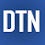## Pages

### Multi-output Regression Example with Keras Sequential Model

Multi-output regression data contains more than one output value for a given input data. We can easily fit and predict this type of regression data with Keras neural networks API. In this tutorial, we'll learn how to fit multi-output regression data with Keras sequential model in Python. The post covers:
1. Preparing the data
2. Defining the model
3. Predicting and visualizing the result
4. Source code listing

```from keras.models import Sequential
from keras.layers import Dense
from numpy import array
from numpy.random import uniform
from numpy import hstack
import matplotlib.pyplot as plt
from sklearn.model_selection import train_test_split
from sklearn.metrics import mean_squared_error```

Preparing the data

Data preparation is an import part of this tutorial. Once you can prepare your data in a correct format, the simple sequential model can handle the remaining part of the problem. We'll create a multi-output dataset for this tutorial. It is randomly generated data with some rules. You can check the logic of data generation in the below function. There are three inputs and two outputs in this dataset.

```def create_data(n):
x1 = array([i/100+uniform(-1,3) for i in range(n)]).reshape(n,1)
x2 = array([i/100+uniform(-3,5)+2 for i in range(n)]).reshape(n,1)
x3 = array([i/100+uniform(-6,5)-3 for i in range(n)]).reshape(n,1)

y1= [x1[i]-x2[i]+x3[i]+uniform(-2,2) for i in range(n)]
y2= [x1[i]+x2[i]-x3[i]+5+uniform(-1,3) for i in range(n)]
X = hstack((x1, x2, x3))
Y = hstack((y1, y2))
return X, Y
```

Now, we'll set n sample numbers and generate the dataset. We can visualize X or Y data in a plot.

```X, Y = create_data(n=450)

plt.plot(Y)
plt.show()```

We'll extract the input and output dimensions from the shape of X and Y data and keep them to use in a keras model below.

`print("X:", X.shape, "Y:", Y.shape)`
`X: (450, 3) Y: (450, 2)`
` `
```in_dim = X.shape
out_dim = Y.shape```

Next, we'll split the data into the train and test parts.

`xtrain, xtest, ytrain, ytest=train_test_split(X, Y, test_size=0.15)`

Defining the model

We'll define a sequential model and fit it with the train data. The sequential model contains Dense layers with ReLU activations and Adam optimizer. Here, an important part of the model definition is the setting of the input dimension in the first layer and output dimension in the last layer.

```model = Sequential()
```
` `
`model.summary()`
```_________________________________________________________________
Layer (type)                 Output Shape              Param #
=================================================================
dense_334 (Dense)            (None, 100)               400
_________________________________________________________________
dense_335 (Dense)            (None, 32)                3232
_________________________________________________________________
dense_336 (Dense)            (None, 2)                 66
=================================================================
Total params: 3,698
Trainable params: 3,698
Non-trainable params: 0
_________________________________________________________________```

We'll fit the model with train data.

`model.fit(xtrain, ytrain, epochs=100, batch_size=12, verbose=0)`

Predicting and visualizing the result

Finally, we'll predict test data and check to mean squared error rate.

```ypred = model.predict(xtest)
print("y1 MSE:%.4f" % mean_squared_error(ytest[:,0], ypred[:,0])) ```
`print("y2 MSE:%.4f" % mean_squared_error(ytest[:,1], ypred[:,1]))`
` `
`y1 MSE:1.4581`
`y2 MSE:1.6905 `

The results can be visualized as below.

```x_ax = range(len(xtest))
plt.scatter(x_ax, ytest[:,0],  s=6, label="y1-test")
plt.plot(x_ax, ypred[:,0], label="y1-pred")
plt.scatter(x_ax, ytest[:,1],  s=6, label="y2-test")
plt.plot(x_ax, ypred[:,1], label="y2-pred")
plt.legend()
plt.show()```

In this tutorial, we've briefly learned how to fit and predict multi-output regression data with keras sequential model. The full source code is listed below.

Source code listing

```from keras.models import Sequential
from keras.layers import Dense
from numpy import array
from numpy.random import uniform
from numpy import hstack
import matplotlib.pyplot as plt
from sklearn.model_selection import train_test_split
from sklearn.metrics import mean_squared_error

def create_data(n):
x1 = array([i/100+uniform(-1,3) for i in range(n)]).reshape(n,1)
x2 = array([i/100+uniform(-3,5)+2 for i in range(n)]).reshape(n,1)
x3 = array([i/100+uniform(-6,5)-3 for i in range(n)]).reshape(n,1)

y1= [x1[i]-x2[i]+x3[i]+uniform(-2,2) for i in range(n)]
y2= [x1[i]+x2[i]-x3[i]+5+uniform(-1,3) for i in range(n)]
X = hstack((x1, x2, x3))
Y = hstack((y1, y2))
return X, Y

X, Y = create_data(n=450)

plt.plot(Y)
plt.show()

print("X:", X.shape, "Y:", Y.shape)
in_dim = X.shape
out_dim = Y.shape

xtrain, xtest, ytrain, ytest=train_test_split(X, Y, test_size=0.15)
print("xtrain:", xtrain.shape, "ytrian:", ytrain.shape)

model = Sequential()
`model.summary()`
` `
`model.fit(xtrain, ytrain, epochs=100, batch_size=12, verbose=0)`

` `
```ypred = model.predict(xtest)
print("y1 MSE:%.4f" % mean_squared_error(ytest[:,0], ypred[:,0]))
print("y2 MSE:%.4f" % mean_squared_error(ytest[:,1], ypred[:,1]))

x_ax = range(len(xtest))
plt.scatter(x_ax, ytest[:,0],  s=6, label="y1-test")
plt.plot(x_ax, ypred[:,0], label="y1-pred")
plt.scatter(x_ax, ytest[:,1],  s=6, label="y2-test")
plt.plot(x_ax, ypred[:,1], label="y2-pred")
plt.legend()
plt.show()```

1.Is it possible to predict more than 2 parameters in a regression problem?

1.You can test it by adding y3 label data.

2.3.Is there a way to see the specific predicted outputs from a new set of inputs?

4.how can I change the dimension of X1 and Y1

5.Can you provide an example for a multi output regression using data generators?